Courses

# Study Notes for Fourier Series Representation of Continuous Periodic Signals -1 Electrical Engineering (EE) Notes | EduRev

## Electrical Engineering (EE) : Study Notes for Fourier Series Representation of Continuous Periodic Signals -1 Electrical Engineering (EE) Notes | EduRev

The document Study Notes for Fourier Series Representation of Continuous Periodic Signals -1 Electrical Engineering (EE) Notes | EduRev is a part of the Electrical Engineering (EE) Course Signal and System.
All you need of Electrical Engineering (EE) at this link: Electrical Engineering (EE)

Fourier Series Representation
The Periodic functions are the functions which can define by relation f(t + P) = f(t) for all t.For example, if f(t) is the amount of time between sunrise and sunset at a certain latitude, as a function of time t, and P is the length of the year, then f(t + P) = f(t) for all t, since the Earth and Sun are in the same position after one full revolution of the Earth around the Sun.

Fourier Theorem

• We will first state Fourier’s theorem for periodic functions with period P = 2π. In words, the theorem says that a function with period 2π can be written as a sum of cosines and sines which all have period 2π.

Suppose f(t) has period 2π then we have
f(x) ≅  a0/2 +{a1cos(x) + a2cos(2x) + a3cos(3x)}+ ........+{b1sin(x) + b2sin(2x) + b3sin(3x)}+ ...
Complete Fourier series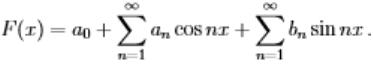-------(1)

• where a0, a1,... and b1, b2 . . . called Fourier coefficients & are computed as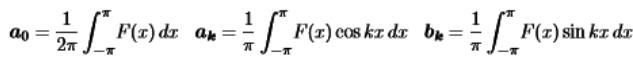• The above is called the Fourier series for the function.That is, sines and cosines, the simplest periodic functions, are the “building blocks" for more general periodic functions.
• There are some terminology coming from acoustics and music: the n = 1 called the fundamental, and the frequencies n ≥ 2 are called the higher harmonics.
• cos(nt) and sin(nt) are periodic on the interval 2π for any integer n. The an and bn coefficients measure the strength of contribution from each "harmonic"

Fourier Sine Series
The Fourier Sine series can be written as
S(x) = b1 sinx + b2 sin 2x + b3 sin 3x +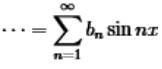........(2)

• Sum S(x) will inherit all three properties: (i): Periodic S(x +2π)=S(x);  (ii): Odd S(−x)=−S(x);    (iii):  S(0) = S(π)=0
• Our ﬁrst step is to compute from S(x) the number bk that multiplies sinkx.

Suppose S(x)=∑ bn sinnx. Multiply both sides by sin kx. Integrate from 0 to π in Sine Series in equation (2)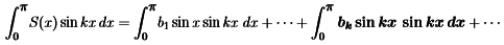• On the right side, all integrals are zero except for n = k. Here the property of “orthogonality” will dominate. The sines make 90o angles in function space, when their inner products are integrals from 0 to π.
• Orthogonality for sine Series
Orthogonality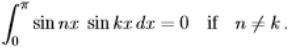.........(3)
• Zero comes quickly if we integrate the term comx  from 0 to π. ⇒ 0π comx dx = 0-0=0
• Integrating cosmx with m = n−k and m = n + k proves orthogonality of the sines.
• The exception is when n = k. Then we are integrating (sinkx)2 = 1/2 − 1/2 cos2kx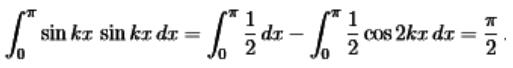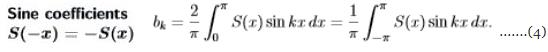• Notice that S(x)sinkx is even (equal integrals from −π to 0 and from 0 to π).
• We will immediately consider the most important example of a Fourier sine series. S(x) is an odd square wave with SW(x) = 1 for 0<x<π. It is an odd function with period 2 π, that vanishes at x=0 and x= π.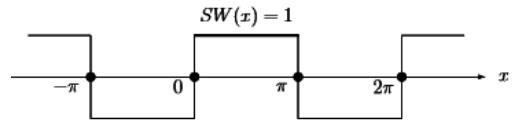Example: Finding the Fourier sine coeﬃcients bk of the square wave SW(x) as given above .
Solution:
For k =1 ,2,...using the formula of sine coefficient with S(x)=1 between 0 and π: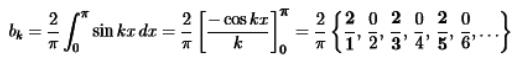• Then even-numbered coeﬃcients b2k are all zero because cos2kπ = cos0 = 1.
• The odd-numbered coeﬃcients bk = 4/πk decrease at the rate 1/k.
• We will see that same 1/k decay rate for all functions formed from smooth pieces and jumps. Put those coeﬃcients 4/πk and zero into the Fourier sine series for SW(x).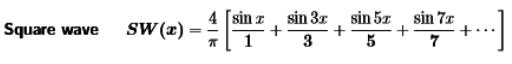Fourier Cosine Series
The cosine series applies to even functions with C(−x)=C(x) as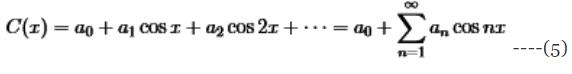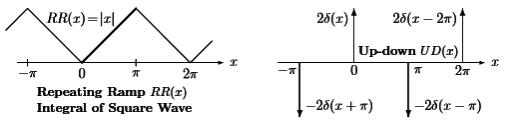Cosine has period 2π shown as above in the figure two even functions, the repeating ramp RR(x) and the up-down train UD(x) of delta functions.

• That sawtooth ramp RR is the integral of the square wave. The delta functions in UD give the derivative of the square wave. RR and UD will be valuable examples, one smoother than SW, one less smooth.
• First we ﬁnd formulas for the cosine coeﬃcients a0 and ak. The constant term a0 is the average value of the function C(x):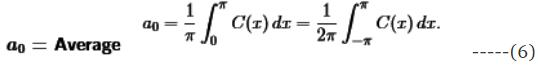• We will integrate the cosine series  from 0 to π. On the right side, the integral of a0 = a0π (divide both sides by π). All other integrals are zero.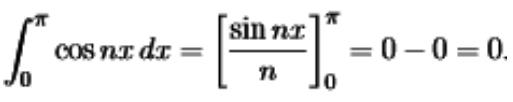• Again the integral over a full period from −π to π (also 0 to 2π) is just doubled.

Orthogonality Relations of Fourier Series
Since from the Fourier Series Representation we concluded that for a periodic Signal it can be written as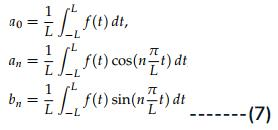The condition of orthogonality as follow: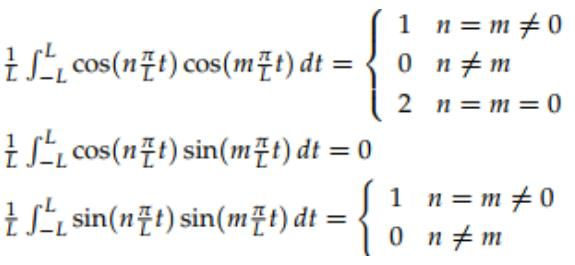Proof of the orthogonality relations: This is just a straightforward calculation using the periodicity of sine and cosine and either (or both) of these two methods:

•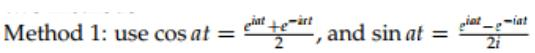• Method 2: use the trig identity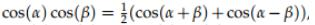and the similar trig identies for cos(α) sin(β) and sin(α) sin(β).

Energy in Function = Energy in Coeﬃcients
There is also another  important equation (the energy identity) that comes from integrating (F(x))2. When we square the Fourier series of F(x), and integrate from −π to π, all the “cross terms” drop out. The only nonzero integrals come from 12 and cos2 kx and sin2 kx, multiplied by a02,ak2 bk2.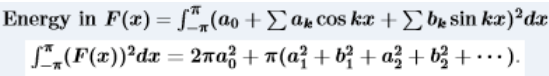• Energy  in F(x) equals the energy in the coeﬃcients.
• Left hand side is like the length squared of a vector, except the vector is a function.
• Right hand side comes from an inﬁnitely long vector of a’s and b’s.
• If the lengths are equal, which says that the Fourier transform from function to vector is like an orthogonal matrix.
• Normalized by constants √2π and √π, we have an orthonormal basis in function space.

Complex Fourier Series

• In place of separate formulas for a0 and ak and bk, we may consider one formula for all the complex coeﬃcients Ck.
• So that  the function F(x) will be complex, The Discrete Fourier Transform will be much simpler when we use N complex exponentials for a vector.

Here we practice in advance with the complex inﬁnite series for a 2π-periodic function:• If every cn = c−n, we can combine einx with e−inx into 2cosnx. Then last equation is the cosine series for an even function.
• If every cn =−c−n, we use einx−e−inx =2i sinnx. Then last equation is sine series for an odd function and the c’s are pure imaginary.
• To ﬁnd ck, multiply (8) by e−ikx (not eikx) and integrate from −π to π.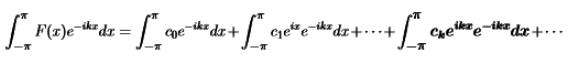• Since the complex exponentials are orthogonal. Every integral on the right side is zero, except for the term (when n = k and (eikx)(e−ikx)= 1). The integral of 1 is 2π.which only term gives the formula for ck.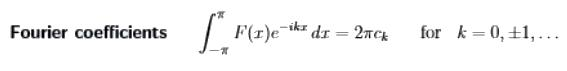• Since c0 = a0 is still the average of F(x), because e0 = 1.
• The orthogonality of einx and eikx is to be checked by integrating.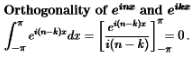Example: Compute the Fourier series of f(t), where f(t) is the square wave with period 2π. which is defined over one period by.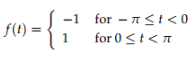The graph over several periods is shown below.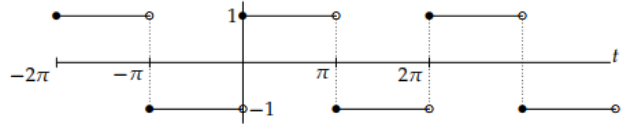Solution: Computing a Fourier series means computing its Fourier coef­ficients. We do this using the integral formulas for the coefficients given with Fourier’s theorem in the previous note. For convenience we repeat the theorem here.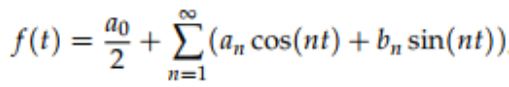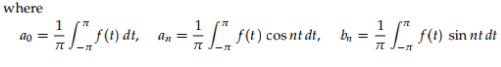By applying these formulas to the above waveform we have to split the integrals into two pieces corresponding to where f(t) is +1 and where it is −1.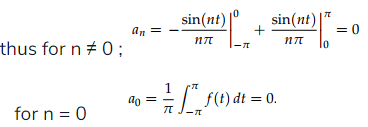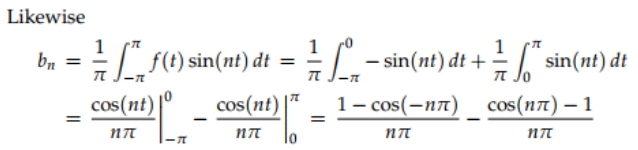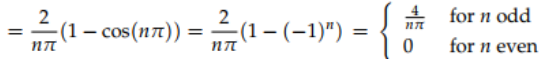We have used the simplification cos nπ = (−1)n to get a nice formula for the coefficients bn.
This then gives the Fourier series for f(t)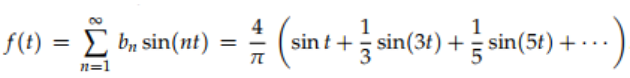Offer running on EduRev: Apply code STAYHOME200 to get INR 200 off on our premium plan EduRev Infinity!

,

,

,

,

,

,

,

,

,

,

,

,

,

,

,

,

,

,

,

,

,

;